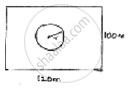Share

# A Park is in the Form of Rectangle 120m × 100m. at the Centre of Park There is a Circular Lawn. the Area of Park Excluding Lawn is 8700m2. Find the Radius of Circular Lawn. - CBSE Class 10 - Mathematics

ConceptAreas of Combinations of Plane Figures

#### Question

A park is in the form of rectangle 120m × 100m. At the centre of park there is a circular lawn. The area of park excluding lawn is 8700m2. Find the radius of circular lawn.

#### SolutionDimensions of rectangular park length = 120m

Area of park = l × b

= 120 × 100 = 12000𝑚2.

𝐿𝑒𝑡 𝑟𝑎𝑑𝑖𝑢𝑠 𝑜𝑓 𝑐𝑖𝑟𝑐𝑢𝑙𝑎𝑟 𝑙𝑎𝑤𝑛 𝑏𝑒 𝑟

Area of circular lawn = 𝜋𝑟2

Area of remaining park excluding lawn = (area of park) – (area of circular lawn)

⇒ 8700 = 12000 – 𝜋r2

⇒ 𝜋r2 = 12000 – 8700 = 3300

⇒22/7× r^2 = 3300

⇒ r^2 = 150 × 7 = 1050

⇒ r = sqrt(1050) = 5sqrt(42) 𝑚𝑒𝑡𝑟𝑒𝑠

∴ radius of circular lawn = 5sqrt(42) metres.

Is there an error in this question or solution?

#### APPEARS IN

Solution A Park is in the Form of Rectangle 120m × 100m. at the Centre of Park There is a Circular Lawn. the Area of Park Excluding Lawn is 8700m2. Find the Radius of Circular Lawn. Concept: Areas of Combinations of Plane Figures.
S